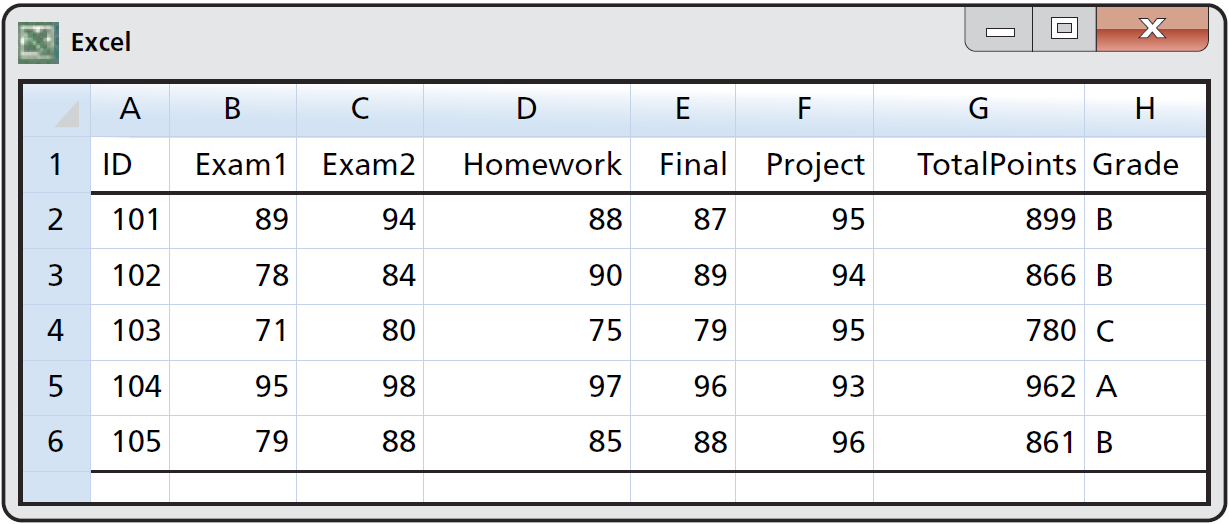×
Log in to StudySoup
Get Full Access to Introduction To The Practice Of Statistics: W/Crunchit/Eesee Access Card - 8 Edition - Chapter 1 - Problem 1.3
Join StudySoup for FREE
Get Full Access to Introduction To The Practice Of Statistics: W/Crunchit/Eesee Access Card - 8 Edition - Chapter 1 - Problem 1.3

Already have an account? Login here
×
Reset your password

# Read the spreadsheet. Refer to Figure 1.2. Give the valuesISBN: 9781464158933 206

## Solution for problem 1.3 Chapter 1

Introduction to the Practice of Statistics: w/CrunchIt/EESEE Access Card | 8th Edition

• Textbook Solutions
• 2901 Step-by-step solutions solved by professors and subject experts
• Get 24/7 help from StudySoup virtual teaching assistantsIntroduction to the Practice of Statistics: w/CrunchIt/EESEE Access Card | 8th Edition

4 5 1 306 Reviews
14
2
Problem 1.3

Read the spreadsheet. Refer to Figure 1.2. Give the values of the variables Exam1, Exam2, and Final for the student with ID equal to 104.

Step-by-Step Solution:

Problem 1.3

Read the spreadsheet. Refer to Figure 1.2. Give the values of the variables Exam1, Exam2, and Final for the student with ID equal to 104.Step by step solution:

Step 1:

From the spreadsheet, the values of the variables of the student with ID equal to 104 are,

Exam1 = 95

Exam2 = 98

Final = 96

These values correspond to the variables in the row with ID equal to 104.

Step 2 of 1

##### ISBN: 9781464158933

Unlock Textbook Solution

Enter your email below to unlock your verified solution to:

Read the spreadsheet. Refer to Figure 1.2. Give the values